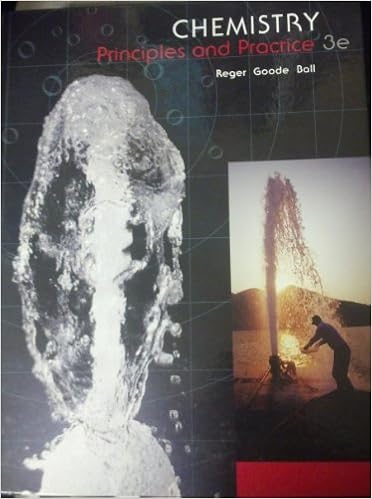# Determine the electron geometry eg and molecular

• Test Prep
• 8
• 100% (3) 3 out of 3 people found this document helpful

This preview shows page 3 - 5 out of 8 pages.

##### We have textbook solutions for you!
The document you are viewing contains questions related to this textbook.The document you are viewing contains questions related to this textbook.
Chapter 8 / Exercise 03
Chemistry: Principles and Practice
Reger ChemExpert Verified
15) Determine the electron geometry (eg) and molecular geometry (mg) of the underlined atom H2CO. A) eg=tetrahedral, mg=tetrahedral B) eg=trigonal planar, eg=trigonal planar C) eg=tetrahedral, mg=bent D) eg=trigonal bipyramidal, mg=tetrahedral E) eg=octahedral, mg=square planar Answer:
B 16) Consider the molecule below. Determine the molecular geometry at each of the 2 labeled carbons. A) C1 = tetrahedral, C2 = linear B) C1 = trigonal planar, C2= bent C) C1 = bent, C2 = trigonal planar D) C1 = trigonal planar, C2 = tetrahedral E) C1 = trigonal pyramidal, C2 = see-saw
D 17) Consider the molecule below. Determine the molecular geometry at each of the 3 labeled atoms.
##### We have textbook solutions for you!
The document you are viewing contains questions related to this textbook.The document you are viewing contains questions related to this textbook.
Chapter 8 / Exercise 03
Chemistry: Principles and Practice
Reger ChemExpert Verified
E) 1=trigonal planar, 2=trigonal pyramidal, 3=trigonal pyramidal
A 18) Place the following in order of increasingX-Se-X bond angle, where X represents the outer atoms in each molecule. SeO2SeCl6 SeF2A) SeCl6< SeF2< SeO2 B) SeF2< SeO2 < SeCl6 C) SeF2< SeCl6 < SeO2 D) SeO2< SeF2 < SeCl6 E) SeCl6< SeO2 < SeF2
A 19) Place the following in order of increasingF-A-F bond angle, where A represents the central atom in each molecule. PF3OF2PF4A) PF3< OF2 < PFB) OF2< PF3 < PFC) OF2< PF4< PFD) PF4< OF2< PFE) PF4< PF3 < OF443 3 2
B 20) Place the following in order of decreasingX-A-X bond angle, where A represents the central atom and X represents the outer atoms in each molecule. N2O NCl3NO2A) NCl3> NO2> N2O B) NO2> N2O > NCl3 C) N2O > NO2> NCl3 D) NCl3> N2O > NO2E) N2O > NCl3> NO2
C
•••